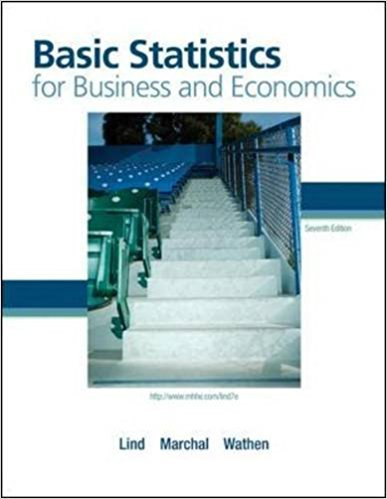×
Get Full Access to Basic Statistics For Business And Economics - 7 Edition - Chapter 3 - Problem 36e
Get Full Access to Basic Statistics For Business And Economics - 7 Edition - Chapter 3 - Problem 36e

×

# Referring to Exercise 43, the annual report of DennisISBN: 9780077384470 64

## Solution for problem 36E Chapter 3

Basic Statistics for Business and Economics | 7th Edition

• Textbook Solutions
• 2901 Step-by-step solutions solved by professors and subject experts
• Get 24/7 help from StudySoup virtual teaching assistantsBasic Statistics for Business and Economics | 7th Edition

4 5 1 377 Reviews
28
0
Problem 36E

Problem 36E

Referring to Exercise 43, the annual report of Dennis Industries also gave these returns on stockholder equity for the same five-year period (in percent): 13.2, 5.0, 10.2, 17.5, and 12.9.

a. What is the arithmetic mean return?

b. What is the variance?

Step-by-Step Solution:

Problem 36E

Referring to Exercise 43, the annual report of Dennis Industries also gave these returns on stockholder equity for the same five-year period (in percent): 13.2, 5.0, 10.2, 17.5, and 12.9.

a. What is the arithmetic mean return?

b. What is the variance?

Step by step solution

Step 1 of 3

The given data X is: 13.2, 5.0, 10.2, 17.5, 12.9.

The number of observations N are 5.

Step 2 of 3

Step 3 of 3

##### ISBN: 9780077384470

Since the solution to 36E from 3 chapter was answered, more than 841 students have viewed the full step-by-step answer. Basic Statistics for Business and Economics was written by and is associated to the ISBN: 9780077384470. This textbook survival guide was created for the textbook: Basic Statistics for Business and Economics , edition: 7. This full solution covers the following key subjects: also, annual, Arithmetic, dennis, equity. This expansive textbook survival guide covers 6 chapters, and 325 solutions. The full step-by-step solution to problem: 36E from chapter: 3 was answered by , our top Business solution expert on 08/23/17, 08:36AM. The answer to “Referring to Exercise 43, the annual report of Dennis Industries also gave these returns on stockholder equity for the same five-year period (in percent): 13.2, 5.0, 10.2, 17.5, and 12.9.a. What is the arithmetic mean return?________________b. What is the variance?” is broken down into a number of easy to follow steps, and 40 words.

Unlock Textbook Solution